# An Analytic Solution for Extraction

In a previous post, we looked at a numerical solution to a model of expresso extraction described by Moroney et al. in their 2019 paper. In this post, I’ll to look at an analytic solution for a simplified version of that model.

You may be asking why we would want an analytic solution. Or you may be wondering what the difference is between a numerical solution and an analytic solution.

We can solve mathematical problems in two basic ways. A numerical solution is an approximate solution expressed in terms of discrete values. An analytic solution, on the other hand, is an exact solution expressed in the language of math. For example, if asked to describe a circle, we could do it either way:

In general, numerical solutions are easier than analytic ones—in many cases, an analytic solution to a mathematical problem isn’t even possible. But where it is possible, it can be descriptive in ways that a numerical solution is not.

I’ll be summarizing the simplified model and analytic solution in this post. If you’re interested in more detail, I’ve also posted the derivation as a Jupyter Notebook on Binder and GitHub.

## The model

For this model, we’re going to divide the espresso puck into two phases: a single solid phase, made up of ground coffee particles; and a liquid phase, made up of water filling the space between coffee particles.

Where water is in contact with the coffee, some soluble compounds move from the location with higher concentration to the one with lower concentration. We describe this using the following equation: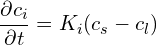The movement of soluble compounds across the boundary depends on two things:

• A constant,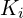describing how easy it is to move across the boundary
• The difference in concentration,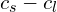, across the boundary

The other important factor in espresso extraction is the flow of water through the puck. Fresh water enters at the top of the puck, moves through it picking up soluble compounds, and eventually exits the bottom of the portafilter. This carries away soluble compounds and keeps the concentration of soluble coffee in the water lower than it would be without flow.

Mathematically, we describe the flow of water through the puck like this:Here,is the velocity of the liquid phase. If we put these two equations together, we get expressions for the change in concentration of soluble coffee in both phases: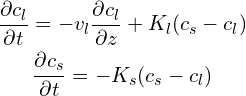The constants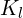and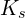describe the same thing—how easily soluble coffee moves between the solid and liquid phases—but in two different frames of reference. We can define these in terms of fundamental properties of the ground coffee as follows: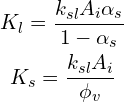Here,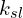is the mass transfer coefficient between the solid and liquid phases,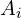is the surface area per unit volume for the coffee particles,is the volume fraction of the solid phase, and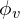is the intragranular porosity of the coffee particles.

## Describing a single layer

To make it easier to find a solution, we describe the puck as a single layer using discrete coordinates located as shown here:

Using this set of coordinates, we can replace the spatial derivative using a backward finite difference: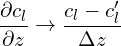We also replace references to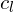with a measurement centered in the puck. This gives the average concentration within the layer.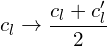Finally, we set a boundary condition,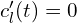. This says that the water entering the top of the puck is clean. With these changes, we get the following set of homogeneous differential equations: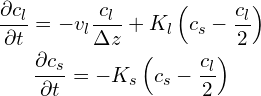## The solution

We can solve these equations using the method of elimination. We define a few constants to keep things clean:and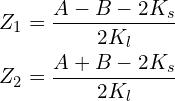With these definitions, the solution is as follows: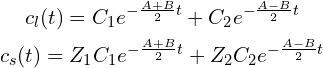## Initial conditions

Any value of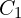and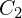will give a valid solution to our system of differential equations. To find the value of these two constants corresponding with a particular situation, we look at what happens when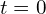: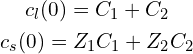We can solve this forand: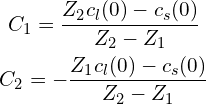With the addition ofand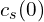—the initial concentrations in the liquid and solid phases—we have a completely specified analytic solution for our system of differential equations.

## Comparison with the numerical solution

Next, let’s compare this solution with the numerical solution of Moroney et al. We will compare with their finely ground single grain model (i.e., using only one size of particle in the solid phase), as well as with their experimental results.

Here, we’ve used the same parameters specified in the paper. Moroney et al. optimized the mass transfer coefficient to match the experimental data. If we do the same, rather than using their reported value, we get an even better fit:

It’s astonishing that our analytic solution matches the numerical solution so well, considering that it treats the packed bed as a single layer, whereas the numerical model treats it as several layers. This suggests that, in terms of modeling average extraction yield, it is not critical to consider the individual layers.

## Transient and steady state behaviour

As an example of the descriptive power of an analytic solution, let’s look at the transient and steady state behaviour of our system. As it turns out, we can split the solution presented above into two parts: one which plays a role early in the shot, but dies out quickly, and another which is dominant later in the shot. This is fairly clear when we look at the form of our solution: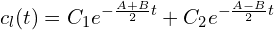Since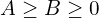, we know that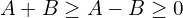, and so the first term must vanish faster than the second term. We can express these two terms as separate solutions:where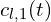is the transient solution, and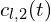is the steady state solution. We can plot these separately to get a more intuitive idea of how they contribute to the overall solution:

Here, we can see that the steady state solution encodes the simple notion of extraction that we discussed in our previous post, while the transient solution acts as a sort of correction to bring the model in line with initial conditions. I suspect—but it may be difficult to prove—that most extractions with a constant flow rate will follow a curve very close to the steady state solution.

## Conclusion

Looking ahead, I’d like to use the analytic model to fit the extraction data I’ve been gathering.

We can eliminate a couple of variables by measuring the volume fraction of coffee particles,, and the intragranular porosity,, directly. I believe we can do this using data from gas pycnometry.

Then, we can optimize to find the model parameters which best fit experimental data. With this, extraction kinetics would be defined in terms of one parameter inherent to the ground coffee (), one inherent to the extraction process (), and one defining initial conditions (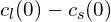).

1.Doron Rainish says:

Hi Michael,
I have used your model in a paper called “Optimal Water Flow Profile in Espresso Coffee Extraction” now published in ResearchGate as open access. I believe you will find it interesting as it may be another small step in the way to quantitative café.
Best regards,
Doron

1.Michael Cooper says:

I love what you’ve done with this. It’s going to take me a while to go through your paper in detail, but initially I’m very intrigued to see that the optimization for Type I extractions has yielded separate “wetting”, “charging”, “extracting”, and “discharging” phases in the extraction process.

In Figure 12, you show a comparison between the optimal flow vs. flat flow. Do you have a sense of how much of this difference comes from the addition of a charging state, vs. how much comes from the discharging state? I’m curious about the relative importance of these two states in the optimization.

2.Doron Rainish says:

Hi Michael,
A good question. The answer can be easily calculated but I believe that the “charge” phase is more important. Looking at Fig. 3, The liquid concentration Cl (red line) is growing very fast at this phase (first ~8 seconds) when the water is being charged with coffee extractions. The “discharge” phase goal to my understanding is to balance the flow rates and the timing of the two first phases. If one skips the charge phase, the discharge phase will be meaningless, probably counter-productive.
Best regards,
Doron

1.sprogeeks says:

Doron Rainish / Hi Doron, I’m not sure what exactly you mean by the discharging process in your paper.

It looks like you were using an e61 espresso machine to conduct your tests, what exactly do you mean by discharging?

1.Doron Rainish says:

Hi,
First of all, I would like to make clear that although the model used in the paper (Moroney, reference ) was validated by experiment, the paper itself is entirely theoretic. I used E61 parameters but did not run any tests. The “discharge” phase is a result of the optimal control theory used on this model.
My understanding is that the “charging” and the “high extraction efficiency” phases are the most important ones, where most of the extraction is done. However, in order to balance between the durations of these phases and the flow rate in the “high extraction efficiency” phase, on one hand, and the constraints of extraction time the water volume on the other hand, the optimal control solution chooses to use an additional degree of freedom which is the “discharging” phase.
From a practical point of view, since this phase is typically very short, and home espresso machine, and even a coffeeshop espresso machine pump may not be able to jump that fast from one flow rate to another, it looks like that this phase could be beneficial to industry level of machines only.
Best regards
Doron

1.Lee Sang Sun says:

I kind of get it, I guess the discharge behavior would be more aggressive if there was an air driven espresso machine instead of a regular espresso machine. I’ll read your paper again.